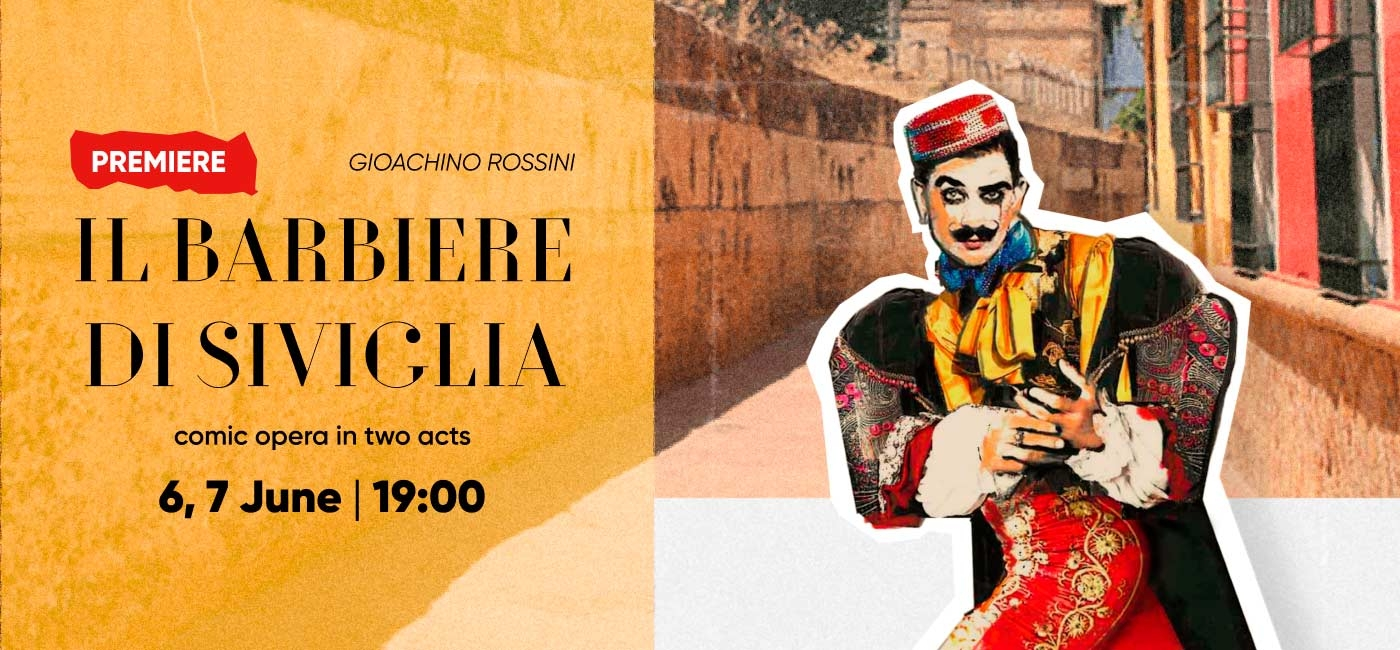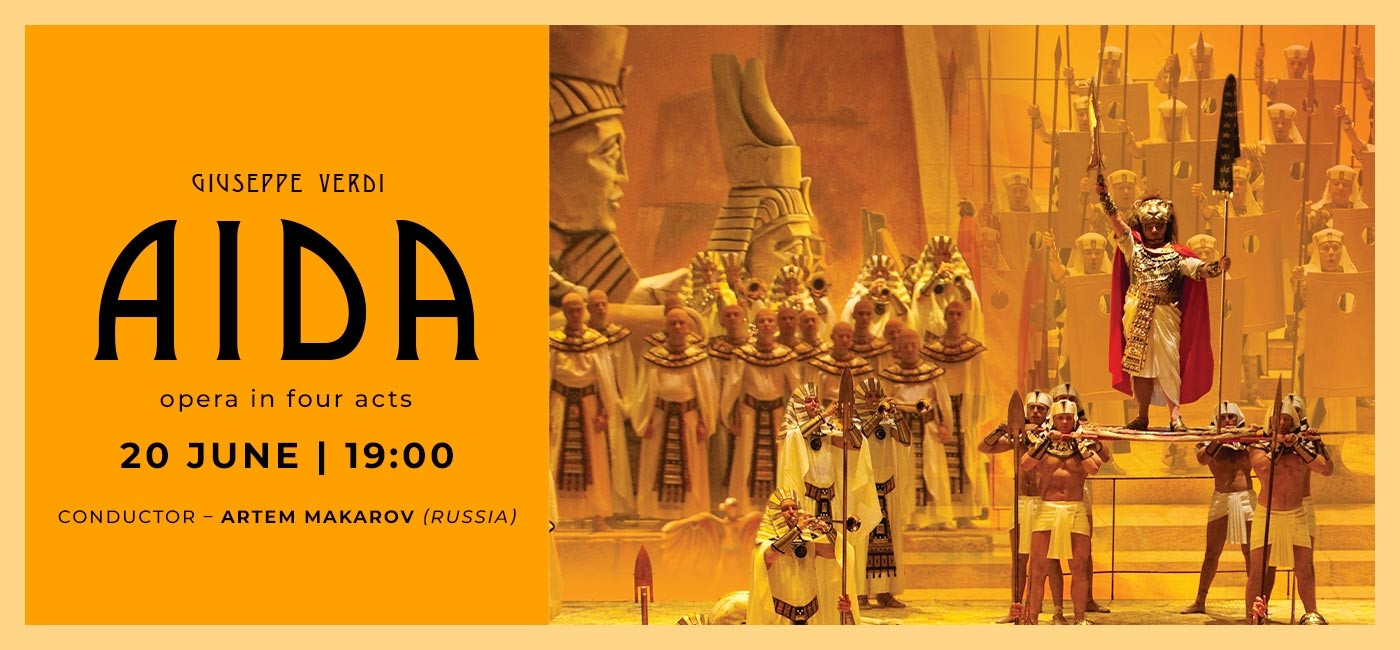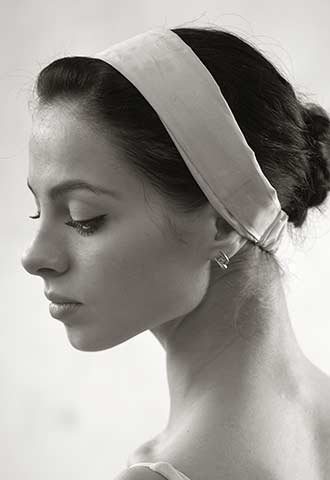# Aleksandra Chizhik

### ScheduleFrancysk Skaryna Medal (2017)
Third prize at the 3rd International Ballet Competition in Astana (Kazakhstan, 2016)
Laureate of the Special Fund of the President of the Republic of Belarus for Support of Talented Youth
Silver medal at the 6th Open Youth Delphic Games of the CIS Member States (Armenia, 2010)

Aleksandra Chizhik graduated from the Belarusian State Choreographic College.

She joined the ballet company of the National Academic Bolshoi Opera and Ballet Theatre of the Republic of Belarus in 2010.

In 2017 she was awarded grand prize and the title of a laureate of the Special Fund of the President of the Republic of Belarus for Support of Talented Youth.

### REPERTOIRE

Anna KareninaВ  В  В  В  В  В  В  В  В  В  В  В  В  В  В  В  В  В  В  В  В  В  В  В  В  В  В В Anna Karenina
MashaВ В В В В В В В В В В В В В В В В В В В В В В В В В В В В В В В В В В В В В В В В В В В В В В В В В В В В В В В В В В В В В В В  The Nutcracker, or Another Christmas Story
ZaremaВ В В В В В В В В В В В В В В В В В В В В В В В В В В В В В В В В В В В В В В В В В В В В В В В В В В В В В В В В В В В В В  The Fountain of Bakhchisarai
Kitri, Mercedes, Street Dancer and DulcineaВ  В  В  В  В  В В Don Quixote
Gamzatti, pas d'actionВ  В  В  В  В  В  В  В  В  В  В  В  В  В  В  В  В  В  В  В  В La BayadГЁre
Variations,Grand PasВ  В  В  В  В  В  В  В  В  В  В  В  В  В  В  В  В  В  В  В  В  В В Paquita
The title role В В В В В В В В В В В В В В В В В В В В В В В В В В В В В В В В В В В В В В В В В В В В В В В В В В В В В  La Esmeralda
The Lilac Fairy, AuroraВ  В  В  В  В  В  В  В  В  В  В  В  В  В  В  В  В  В  В  В  В The Sleeping Beauty
Odette/Odile, pas de troisВ  В  В  В  В  В  В  В  В  В  В  В  В  В  В  В  В  В  В Swan Lake В
The Angel В В В В В В В В В В В В В В В В В В В В В В В В В В В В В В В В В В В В В В В В В В В В В В В В В В В В В В В В В  The Creation of the World
Russian DanceВ В В В В В В В В В В В В В В В В В В В В В В В В В В В В В В В В В В В В В В В В В В В В В В В В В В  Serenade
Laurencia, Jacinta, PascualaВ  В  В  В  В  В  В  В  В  В  В  В  В  В  В  В  В Laurencia
The Beloved В В В В В В В В В В В В В В В В В В В В В В В В В В В В В В В В В В В В В В В В В В В В В В В В В В В В В  Le Petit Prince
The Fairy of Dance,В Stepsister (Krivlyaka)В  В  В  В  В  В  В Cinderella
Dance of Three SylphsВ  В  В  В  В  В  В  В  В  В  В  В  В  В  В  В  В  В  В  В В La Sylphide
Medora, Gulnare, dance of three OdalisqueВ  В  В  В  В  В Le Corsaire
MyrthaВ  В  В  В  В  В  В  В  В  В  В  В  В  В  В  В  В  В  В  В  В  В  В  В  В  В  В  В  В  В  В В Giselle
The HarlotВ  В  В  В  В  В  В  В  В  В  В  В  В  В  В  В  В  В  В  В  В  В  В  В  В  В  В  В  В Carmina Burana
The title role, the GypsyВ  В  В  В  В  В  В  В  В  В  В  В  В  В  В  В  В  В  В В Anyuta
The WomanВ  В  В  В  В  В  В  В  В  В  В  В  В  В  В  В  В  В  В  В  В  В  В  В  В  В  В  В Orr and Ora
JadwigaВ  В  В  В  В  В  В  В  В  В  В  В  В  В  В  В  В  В  В  В  В  В  В  В  В  В  В  В  В  В  В Vitaut
Russian, Spanish, French danceВ  В  В  В  В  В  В  В  В  В  В  В  В  В The Nutcracker
Zobeide, Dance of Three SoloistsВ  В  В  В  В  В  В  В  В  В  В  В  В Shaherezada
SoloistВ  В  В  В  В  В  В  В  В  В  В  В  В  В  В  В  В  В  В  В  В  В  В  В  В  В  В  В  В  В  В В Sechs tГ¤nze
The Byzantine BeautyВ В В В В В В В В В В В В В В В В В В В В В В В В В В В В В В В В В В В В В В  Seven Beauties
ZairaВ  В  В  В  В  В  В  В  В  В  В  В  В  В  В  В  В  В  В  В  В  В  В  В  В  В  В  В  В  В  В  В В Anastasia
The title roleВ  В  В  В  В  В  В  В  В  В  В  В  В  В  В  В  В  В  В  В  В  В  В  В  В  В В The Firebird
SoloistВ  В  В  В  В  В  В  В  В  В  В  В  В  В  В  В  В  В  В  В  В  В  В  В  В  В  В  В  В  В  В La Petite Mort
GrisiВ  В  В  В  В  В  В  В  В  В  В  В  В  В  В  В  В  В  В  В  В  В  В  В  В  В  В  В  В  В  В  В В Pas de Quatre

В В

### Partners